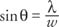## Diffraction

Thomas Young's double‐slit experiment shows that light spreads out in wavefronts that can interfere with each other. Diffraction is the effect of a wave spreading as it passes through an opening or goes around an object. The diffraction of sound is quite obvious. It is not at all remarkable to hear sound through an open door or even around corners. In contrast, diffraction is quite difficult to observe with light. The difference is that sound waves are long while light waves are extremely short because differentiation is proportional to wave length; it is not easy to observe the bending of light when it passes through a small aperture or goes around a sharp edge.

A single slit yields an interference pattern due to diffraction and interference. Imagine that the slit is wide enough to allow a number of wavelets. Figure 1 shows the wave‐ray diagram used to analyze the single slit.Figure 1 Diffraction of light through a single slit.

The rays from A and B interfere at P on a distant screen. As shown, AP exceeds BP by half a wavelength; therefore, the represented waves destructively interfere. Also for every wave originating between A and B, there is another point between B and C with a wavelet that will destructively interfere. The wavelets cancel in pairs; thus, point P is a minimum or dark point on the screen.

The triangle ACD is nearly a right triangle if P is quite distant. Applying the definition for sine to the figure yieldswhere λ is the wavelength and w is the slit width. Whenever the path difference between AP and CP is a whole number of wavelengths, a dark fringe will be produced on the screen because the wavelets can be seen to completely cancel in pairs.

Figure 2 illustrates the light rays traveling to another point on the screen.Figure 2 Diffraction of light through a single slit.

In this case,The region of wavelets is divided into three. Again, the waves through two regions cancel in pairs, but now the waves from one region constructively interfere to produce a bright point on the screen. This is partial reinforcement. The positions of the light and dark fringes formed by a single slit are summarized in the intensity versus angle sketch shown in Figure 3. The center region of the pattern will be the brightest band because the wavelets completely, constructively interfere in the middle.Figure 3 Position of fringes produced by single-slit diffraction.

When looking through double slits, it is impossible to see only the double‐slit pattern because the double‐slit is really two single slits; therefore, the actual observed pattern is that of superimposed double – and single‐slit patterns.